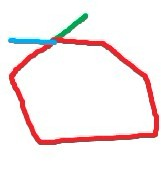# 【CSON原创】HTML5圈泡泡游戏发布

在一分钟内，使用鼠标按着左键，在画布上圈泡泡，其中泡泡的分值分别为10（白）、20（浅蓝）、30（黄）、-10（红）、-20（绿）、-30（深蓝）分，可以一次圈多个泡泡，倒计时结束即计算总分值，该游戏基于cnGameJS

首先每个小球定义一个ball类，由于小球需要使用图片，并且有一定的尺寸和运动，所以使该类继承cnGameJS的sprite类。ball类除了拥有x,y坐标外，还拥有一个z坐标，该坐标用于使小球具有离玩家远近的视觉差。
/*    小球对象    */
var Ball=function(opt){
this.parent.call(this,opt);
this.oriPos=[this.x+this.width/2,this.y+this.height/2];
this.oriSize=opt.size;
this.z=opt.z||0;
this.score=opt.score||0;
this.oriSpeedZ=4+Math.random()*4;
this.scale=1;
this.resetXY();

}
cg.core.inherit(Ball,Sprite);
之后我们为小球添加resetXY方法，该方法根据小球的z坐标，改变小球的位置以及尺寸，使小球有远近的视觉差。首先根据z计算缩放比scale，然后根据scale调整x,y和width,height，另外我们使小球z大于1000时，小球消失，这样就避免了小球过大而占据整个屏幕。
cg.core.extendProto(Ball,{
disappear:function(){//小球被选中消失
list.remove(this);
},
resetXY:function(){//根据Z改变x,y的位置和尺寸
var oriX=this.oriPos;
var oriY=this.oriPos;
var oriSize=this.oriSize;
this.scale=((center+this.z)/center);//相对于现时的scale
this.x=(oriX-center)*this.scale+center;
this.y=(oriY-center)*this.scale+center;
this.height=this.width=this.oriSize*this.scale;
this.speedZ=this.oriSpeedZ*this.scale;
if(this.z>1000){
this.disappear();
}
},
update:function(){
this.parent.prototype.update.call(this);
this.resetXY();
}
});

之后，为了管理多个小球，可以增加一个小球管理器。管理器负责动态改变小球到玩家的距离以及使小球在画布随机的位置出现：

/*    小球对象管理器    */
var ballsManager={
createDuration:200,
ballSize:30,
lastCreateTime:Date.now(),
/*    随机生成小球    */
createRandomBalls:function(num){
var now=Date.now();
if(now-this.lastCreateTime>this.createDuration){
for(var i=0;i<num;i++){
var x=Math.random()* cg.width;
var y=Math.random()* cg.height;
var randomKind=ballKinds[Math.floor(Math.random()*6)];//随机获得的小球种类和分值
var newBall=new Ball({x:x,y:y,size:this.ballSize,z:-280,score:randomKind});
newBall.setCurrentImage(srcObj[randomKind]);//设置图片
}
this.lastCreateTime=now;
}
},
/*    改变小球位置    */
changeBallsPos:function(){
var ballsArr=list.get(function(elem){
return elem instanceof Ball;
});
for(var i=0,len=ballsArr.length;i<len;i++){
var ball=ballsArr[i];
ball.z+=ball.speedZ;
}
}
}

关于小球管理就介绍到这里，之后主要介绍怎样实现鼠标的圈选。
如果我们在每次帧更新时，根据鼠标现时的位置以及上一次的位置绘制一条线段，那么鼠标的移动轨迹就可以被一条曲线表示出来，该曲线由每次绘制的线段组成，因此我们也可以说该曲线是一条由多条线段首尾相连组成的曲线。因此我们可以首先实现一个线段类：

        /*    直线    */
var line=function(options){
if (!(this instanceof arguments.callee)) {
return new arguments.callee(options);
}
this.init(options);
}

line.prototype = {
/**
*初始化
**/
init: function(options) {
this.start=[0,0];
this.end=[0,0];
this.style="red";
this.lineWidth=1;
this.context=cg.context;
options = options || {};
cg.core.extend(this,options);
},
该类保存线段的起始点坐标和结束点坐标，以及宽度，样式等。

之后需要考虑的就是怎样实现圈选了。当我们用鼠标画出一个圈时，每条小线段就组成了一个闭合的多边形，这时我们就可以说鼠标圈出了一个闭合区域，之后就可以进一步计算哪些小球在该区域里面。

但是怎样判断鼠标是否圈出了一个闭合区域呢？这里使用的方法是：遍历每一条线段，从该线段的下一条再下一条线段开始遍历余下的线段，判断它们中是否有线段和开始的那条线段相交，如果相交，则证明曲线闭合了。注意这里从线段的下条再下条线段开始遍历是为了跳过线段首尾相连的情况。（例如，第一条线段肯定和第二条线段相交，因此从第三条线段开始判断，跳过相邻线段收尾相交的情况），代码如下：

    /*    返回轨迹是否闭合    */
var isClose=function(lines){
var hasClose=false;
for(var i=0;i<lines.length;i++){
var l1=lines[i];
for(var j=i+2;j<lines.length;j++){
var l2=lines[j];
if(l2){
var point=l1.isCross(l2);//交点坐标
if(point){//非连接的相交
resetLineSegs(lines,i,j,point);
hasClosed=true;
return true;
}
}
}
}

return false;
};                  

isCross方法返回线段交点的坐标,我们获得该坐标后，还需要把多边形修正成为真正的多边形，因为用鼠标圈出来的多边形并不是一个真正的多边形，它的开始和结束部分很可能会有突出的地方，如下图：我们假设鼠标从绿色部分开始圈一个圈，到蓝色部分结束。这样的话轨迹就并不是一个严格的多边形，因为它多出了蓝色和绿色的部分。因此我们需要对圈出来的多边形进行一个修正操作，使其变成一个真正的闭合多边形：

    /*    重置线段    */
var resetLineSegs=function(lines,i,j,point){
lines[i].end=point;
lines[i].end=point;
lines[i+1].start=point;
lines[i+1].start=point;

lines[j].start=point;
lines[j].start=point;

lines[j-1].end=point;
lines[j-1].end=point;
for(var m=i+1;m<j;m++){
closedLineSegsArr.push(lines[m]);
}
}
当我们判断到两条线段相交后，可以获取到这两条线段的索引，这里分别为i和j(i<j)，point为交点。在新增加的resetLineSegs方法中，首先改变i线段的结束坐标，使其刚好为交点，再改变j线段的起始坐标，使其为交点，并且使i线段后面的线段的起始坐标以及j线段前面线段的结束坐标为交点，这样就可以使多边形真正闭合。之后把闭合多边形各个边的对象保存在一个数组中，我们后面就要使用这些边，构造多边形对象了。

                        for(var i=0,len=closedLineSegsArr.length;i<len;i++){
pointsArr.push([closedLineSegsArr[i].start,closedLineSegsArr[i].start]);
}
polygon=new Polygon({pointsArr:pointsArr,style:"rgba(241,46,8,0.5)"});
通过多边形的边对象的数组，可以获取到多边形每个顶点的坐标，并根据这些坐标构造多边形对象，之后的工作就是判断小球是否在多边形里面了。
判断小球是否在多边形里，可以转化为判断小球的中点是否在多边形里，这里使用的方法叫射线法，意思是从一点向左发射出一条射线，如果射线和多边形有奇数个交点，则证明点在多边形内部。根据该定理实现的isInside方法如下：
            /**
*判断某点是否在多边形内(射线法)
**/
isInside:function(point){
var lines=this.getLineSegs();

var count=0;//相交的边的数量
var lLine=new Line({start:[point,point],end:[-9999,point]});//左射线
var crossPointArr=[];//相交的点的数组
for(var i=0,len=lines.length;i<len;i++){
var crossPoint=lLine.isCross(lines[i]);
if(crossPoint){
for(var j=0,len2=crossPointArr.length;j<len2;j++){
//如果交点和之前的交点相同，即表明交点为多边形的顶点
if(crossPointArr[j]==crossPoint&&crossPointArr[j]==crossPoint){
break;
}

}
if(j==len2){
crossPointArr.push(crossPoint);
count++;
}

}
}

if(count%2==0){//不包含
return false;
}
return true;//包含
},
另外需要注意的是，由于射线与多边形相交交点个数是通过射线和多边形的每条边是否相交来判断，所以如果射线通过多边形的顶点，我们得出的结果就是相交了两次（通过顶点使射线与两条边都有相交）。因此我们需要记录判断过的交点，每次判断时检查该交点是否已经出现过，若出现过则不纳入计数，这样就基本实现了判断小球是否在鼠标圈选的多边形区域内。
大概就说这么多了，欢迎交流~

demo下载地址：点击下载

posted @ 2012-04-09 20:05  Cson  阅读(3030)  评论(13编辑  收藏